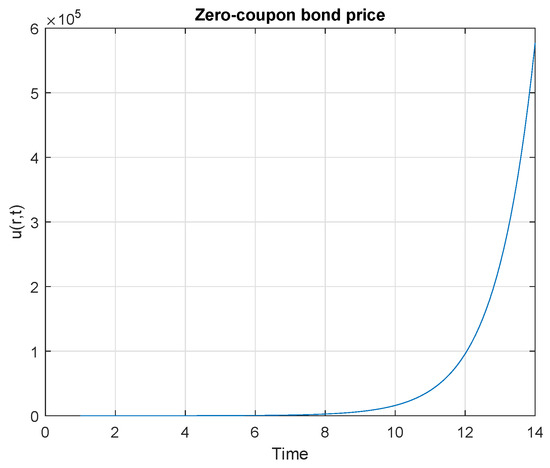Previous Article in Journal
A New Strategy for Improving the Tracking Performance of Magnetic Levitation System in Maglev Train
Open AccessArticle

# Symmetry Analysis of an Interest Rate Derivatives PDE Model in Financial Mathematics

Centre for Computational Finance and Economic Agents, University of Essex, Colchester CO4 3SQ, UK
*
Author to whom correspondence should be addressed.
Symmetry 2019, 11(8), 1056; https://doi.org/10.3390/sym11081056
Received: 23 July 2019 / Revised: 8 August 2019 / Accepted: 12 August 2019 / Published: 16 August 2019
We perform Lie symmetry analysis to a zero-coupon bond pricing equation whose price evolution is described in terms of a partial differential equation (PDE). As a result, using the computer software package SYM, run in conjunction with Mathematica, a new family of Lie symmetry group and generators of the aforementioned pricing equation are derived. We furthermore compute the exact invariant solutions which constitute the pricing models for the bond by making use of the derived infinitesimal generators and the associated similarity reduction equations. Using known solutions, we again compute more solutions via group point transformations. View Full-Text
Show FiguresFigure 1

MDPI and ACS Style

Kaibe, B.C.; O’Hara, J.G. Symmetry Analysis of an Interest Rate Derivatives PDE Model in Financial Mathematics. Symmetry 2019, 11, 1056.

Show more citation formats Show less citations formats
Note that from the first issue of 2016, MDPI journals use article numbers instead of page numbers. See further details here.

1